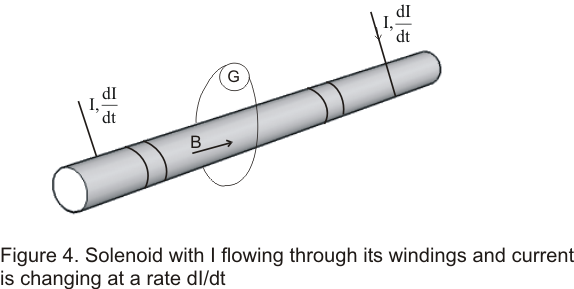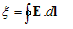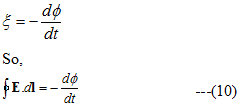# Induced Electric Fields

## Induced Electric Fields

• In the earlier section we have studied that when a conductor moves in a magnetic field induced current is generated
• Now consider a situation in which conductor is fixed in a time varying magnetic field .In this situation magnetic flux through the conducting loop changes with time and an induced current is generated
• Figure below shows a solenoid encircled by a conducting loop with a small galvanometer• Current I through the solenoid sets up a magnetic field B along its axis and a magnetic flux Φ passes through the surface bounded by the loop
• Now when the current I through the solenoid changes ,the galvanometer deflects for the time during which the flux is changing .This indicates that an emf is induced in the conductor.

• From Faraday's law this emf is given by the relation eq Here as we earlier stated that the conductor is stationary and the flux through the loop is changing due to the magnetic field varying on time
• Since charges are at rest (v=0) so magnetic forces Fm=q(v X B) cannot set the charges to motion.Hence induced current in the loop appears because of the presence of an electric field E in the loop
• It is this electric field E which is responsible for the induced emf and hence for the current flowing in a fixed loop placed in a magnetic field varying with time
• This electric field produced here is purely a field of non electrostatic origin i.e it originated due to the magnetic field varying with time, and induced emf may be defined as the line integral of this non-electrostatic field .Thus,• From equation (10) we see that line integral of electric field induced by varying magnetic field differs from zero.This means we can not define a electrostatic potential corresponding to this field.
• Hence this electric field produced by changing electric field is non electrostatics and non-conservative in nature.
• We call such a field as induced electric field.